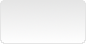# MathJax 3 - Advanced Javascript rendering methods## Introduction

How to render MathJax equations after :

• user input, raw tex code injection

For more interactive content, 2 functions to know using MathJax 3 : typesetPromise, tex2chtmlPromise.

## Content added dynamically after Mathjax has processed the page. typesetPromise, typeset

When content is added dynamically after MathJax has processed a page, how to render the new inserted equations ?

In the example below :

<div class="m-exercice" data-title="Exercise 1">
<div id="exercise-1">Calculate the derivative of $$f(x) = \dfrac{x+2}{x+4}$$</div>
<button id="display-solution-1">Solution</button>
</div>
Calculate the derivative of $$f(x) = \dfrac{x+2}{x+4}$$

When clicking the button "Solution", the display_solution function is called. This function retrieves the solution from an external file using the fetch method (SQLPAC - Javascript : Importing HTML blocks, fetch).

./include/solutions.inc
<div id="solution-1">
\begin{align*} \left (\dfrac{f}{g} \right)' = \dfrac{f'g -g'f}{g^2} \implies f'(x) &= \dfrac{(x+4) - (x+2)}{(x+4)^2} \\ &= \dfrac{2}{(x+4)^2} \end{align*} 
</div>

display_solution function :

b = document.getElementById('display-solution-1');

display_solution = ()=> {
fetch(./include/solutions.inc)
.then ( (r) => { return r.text();  } )
.then ( (s) => {
p= new DOMParser();

d = p.parseFromString(s,'text/html') ;

se1 = d.getElementById('solution-1');

b = document.getElementById('display-solution-1');
b.removeEventListener('click', display_solution);
b.addEventListener('click', () => { render_solution('solution-1'); } );
b.innerHTML='Render';

document.querySelector('main > div[data-title*="Exerci"][data-title\$=" 1"]')
.insertBefore(se1, b);
});
};

The solution is inserted, right, but in raw format, MathJax has already processed the page :

\begin{align*} \left (\dfrac{f}{g} \right)' = \dfrac{f'g -g'f}{g^2} \implies f'(x) &= \dfrac{(x+4) - (x+2)}{(x+4)^2} \\ &= \dfrac{2}{(x+4)^2} \end{align*}

To run MathJax on new added content, use typesetPromise function :

if (window.MathJax) {
node = document.getElementById('solution-1');
MathJax.typesetPromise([node]).then(() => {});
}

If no node is given in argument (array of nodes), the whole page is processed again by MathJax. Nodes already formated are obviously discarded.

In the above example, typesetPromise is performed in the function render_solution, function attached to the button when its caption is changed to "Render" after the solution is retrieved in the DOM.

render_solution = (p)=> {
if (window.MathJax) {
node = document.getElementById(p);
MathJax.typesetPromise([node]).then(() => {
document.getElementById('display-solution-1').style='display:none;';
});
}
};


Obviously in a concrete page, the typesetPromise function is called just after the new content is addded. The user is not required to click on a button "Render".

Another function is available to typeset Math new content : typeset

if (window.MathJax) {
node = document.getElementById(p);
MathJax.typeset([node]);
}

typeset, typesetPromise, what’s the difference ? The first one is used if no extensions/dependencies (require, mhchem, other packages…) have to be auto-loaded to process the block. When extensions may have to be loaded dynamically, the asynchronous typesetPromise function is mandatory.

If Tex/AMS equations autonumbering is active, to reset/recompute equations numbering :

MathJax.texReset([start])

Recomputing equations numbering is not often needed : added contents are usually exercises and so on…, not core demonstrations.

## User input, raw tex code. tex2chtmlPromise

In a textarea field form, the user writes the Tex equation code and clicks on the button "Generate" to get the equation rendering using MathJax :

The form is simple and the resulting MathJax equation rendering is displayed in a div block just below the form :

          <form id="equation-input">
<textarea name="tex-code">\int_{x_0}^{\infty} \frac{x^2}{2} dx</textarea>
<button>Generate</button>
</form>
<div id="equation-result">
</div>

When the DOM is loaded, the form submit action is set to the function display_equation :

display_equation = ()=> {

};

f = document.getElementById('equation-input');
f.addEventListener('submit', (e)=> { e.preventDefault(); display_equation(); } );

In the display_equation function :

• The function MathJax.tex2chtmlPromise is called with the tex code in argument. This function returns in promise the MathJax node result.
• The node is then added to the div block result.
• For some "obscure" reasons, MathJax.startup.document.clear() and MathJax.startup.document.updateDocument() functions must be called at the end.
display_equation = ()=> {
tex = document.querySelector('textarea[name="tex-code"]').value;

d=document.getElementById('equation-result');

MathJax.tex2chtmlPromise(tex).then((node) => {
d.innerHTML='';
d.append(node);
MathJax.startup.document.clear();
MathJax.startup.document.updateDocument();
});
};